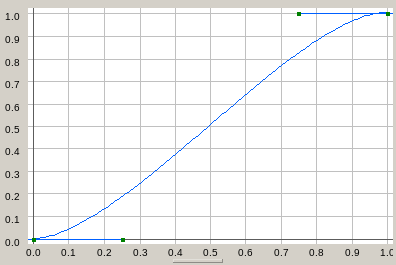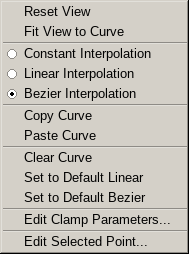#Drag[en]gine Wiki

### Site Tools

gamedev:deigde:editors:widgetbeziercurve

# Bezier Curve Editor

The bezier curve widget is used in various editors to edit bezier curves.Bezier curves are 2D curves mapping an input value to an output value.

Curves compose of a series of curve points ordered by increasing X coordinate. Each curve point composes of three 2-vector values: point, first handle and second handle.

The point defines the location of the curve point (X coordinate, input value) and the output value (Y coordinate).

The two handles define the shape of the curve in the neighbourhood of the point. The first point is located to the left side of the point (leading in handle) and the second handle is located on the right side of the point (leading out handle). If the handles and the point are co-linear (all three vectors can be joined with a continuous line) then the curve has first order continuity (smooth corner). If the distance between the two handles and the point is also identical then the curve has second order continuity (smooth corner and smooth curvature change rate). Otherwise the corner is pointy and has zero order continuity.

For the entire curve the interpolation mode can be set. There are three possible values: constant interpolation, linear interpolation and bezier interpolation.

Constant interpolation forces the output value of each curve segment (the section between two curve points) to the Y-coordinate of the the curve point on the left side of the segment. The value then jumps immediately to the Y-coordinate of the next segment when arriving at the right curve point. In this mode handles are ignored. This mode is best suited for switching type mapping where the output is constant across curve segments. In this mode handles are ignored.

Linear interpolation blends linearly between the left and right end of a curve segment. All corners are pointy. In this mode handles are ignored.

Bezier interpolation produces smooth curves by interpolation using bezier algorithm between curve points.

# View Interaction

The bezier curve editor supports these editing interaction using keyboard and mouse:

• MOUSE_LEFT: Select curve point or handle. Required for certain context menu functions to be enabled.
• MOUSE_LEFT dragging: Move selected curve point or handle. If clamping is enabled and point is moved (not handle) movement is restricted to clamp range. Independent of clamping points can not be moved outside the curve sgement (hence not before the preceding curve point nor past the following curve point). Handles can not be dragged across the point (hence the left handle has to stay on the left side and the right handle has to stay on the right side).
• SHIFT+MOUSE_LEFT: Add curve point.
• SHIFT+MOUSE_LEFT dragging: Add curve point and move it.
• CTRL+SHIFT+MOUSE_LEFT: Delete curve point clicked upon.
• MOUSE_RIGHT: Show context menu

# Context MenuThe context menu allows to edit the curve as well as setting some parameters.

Reset View resets the X-axis and Y-axis to the range 0 → 1.

Fit View to Curve adjusts the X-axis and Y-axis extends to fit the curve into the view. In particular this sets the X-axis range to include the first and last curve point X-coordinate as well as the Y-axis minimum and maximum set to the curve point Y-coordinate minimum respectively maximum value. Handles are not included in the curve fitting only curve points.

Clear Curve removes all curve points.

Set to Default Linear sets the interpolation mode to linear interpolation, removes all curve points and adds the curve points (0,0) and (1,1). Basically this replaces the curve with a linear mapping from (0,0) to (1,1) which keeps the input value unchanged.

Set to Default Bezier sets the interpolation mode to bezier interpolation, removes all curve points and adds two curve points producing an S-shaped curve starting at (0,0) and ending at (1,1). This is similar to a GLSL smooth step curve running from (0,0) to (1,1).

Edit Clamp Parameters shows a dialog to edit the clamping parameters. If clamping is enabled curve points (not handles) are clamped to the desired range while dragged using the mouse. Clamping is not applied to existing points if changed nor to manually edited points.

Edit Selected Point shows a dialog to edit the point, first handle and second handle of the selected curve point.

You could leave a comment if you were logged in.
gamedev/deigde/editors/widgetbeziercurve.txt · Last modified: 2020/03/30 23:51 by dragonlord

### Page Tools